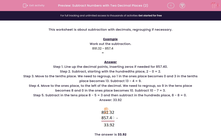# Subtract Numbers with Two Decimal Places (2)

In this worksheet, students subtract numbers with two decimal places.This content is premium and exclusive to EdPlace subscribers.Key stage:  KS 2

Curriculum topic:   Maths and Numerical Reasoning

Curriculum subtopic:   Decimals

Difficulty level:#### Worksheet Overview

This worksheet is about subtraction with decimals, regrouping if necessary.

Example

Work out the subtraction.

891.32 - 857.4 =

Step 1. Line up the decimal points, inserting zeros if needed for 857.40.
Step 2. Subtract, starting with the hundredths place, 2 - 0 = 2.
Step 3. Move to the tenths place. We need to regroup, so 1 in the ones place becomes 0 and 3 in the tenths place becomes 13. Subtract 13 - 4 = 9.
Step 4. Move to the ones place, to the left of the decimal. We need to regroup, so 9 in the tens place becomes 8 and 0 in the ones place becomes 10. Subtract 10 - 7 = 3.
Step 5. Subtract in the tens place 8 - 5 = 3 and then subtract in the hundreds place, 8 - 8 = 0.